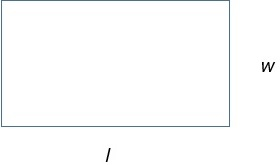# Area of a Square or a Rectangle

#### Complete Python Prime Pack

9 Courses     2 eBooks

#### Artificial Intelligence & Machine Learning Prime Pack

6 Courses     1 eBooks

#### Java Prime Pack

9 Courses     2 eBooks

Area of a plane figure is the measure of space inside the figure and is two dimensional.
It is measured in square units like square inches, square feet, square centimeters or square meters.Formula

If the side of a square is s, the area of the square is given by s2Formula

If the length and width of a rectangle are l and w, then its area

= l × w

If the side of a square is 5 m, find the area of the square

### Solution

Step 1:

The side of the square = 5 m

Step 2:

Then area of the square

(5m)2 = 25 m2

If the length and width of a rectangle are 8 cm and 4 cm, then find its area.

### Solution

Step 1:

The length = 8 cm and the width = 4 cm of the rectangle.

Step 2:

Then its area

= 8 × 4 = 32 square cm